# Primary ideal

## Definition

### Equivalent definitions in tabular format

No. Shorthand An ideal in a commutative unital ring is termed a primary ideal if ... An ideal$I$ in a commutative unital ring is termed a primary ideal if ...
1 product of two elements version it is a proper ideal in the ring and whenever the product of two elements of the ring lies in the ideal, either the first element lies in the ideal or some power of the second element lies in the ideal.$I$ is proper in$R$ and whenever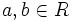$a,b \in R$ (possibly equal, possibly distinct) are such that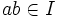$ab \in I$, then either$a \in I$ or there exists a positive integer$n$ such that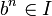$b^n \in I$.
2 exactly one associated prime there is exactly one associated prime to the ideal Fill this in later
3 quotient ring is primary the quotient ring is a primary ring, i.e., it has exactly one associated prime. the quotient ring$R/I$ is a primary ring, i.e., it has exactly one associated prime.
This article defines a property of an ideal in a commutative unital ring |View other properties of ideals in commutative unital rings
This property of an ideal in a ring is equivalent to the property of the quotient ring being a/an: primary ring | View other quotient-determined properties of ideals in commutative unital rings

## Examples

### Important ring types

Property of commutative unital rings Characterization of primary ideals in such a ring Examples
principal ideal domain (i.e., an integral domain in which every ideal is a principal ideal) either the zero ideal or a principal ideal generated by a nontrivial (i.e., use nonzero exponent) power of a prime element . In particular, for a PID, the primary ideals coincide with the powers of prime ideals, and also with the ideals with prime radical. In the ring of integers, the primary ideals are precisely the zero ideal and the ideals generated by powers of primes. Thus,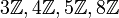$3\mathbb{Z}, 4 \mathbb{Z}, 5\mathbb{Z}, 8\mathbb{Z}$ are primary ideals. On the other hand,$6\mathbb{Z}$ and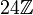$24\mathbb{Z}$ are not primary.
In the (univariate) polynomial ring over a field, the primary ideals are precisely the principal ideals generated by a power of an irreducible polynomial.
Dedekind domain (roughly speaking, unique factorization of ideals into prime ideals) coincides with power of a prime ideal
unique factorization domain the principal primary ideals are precisely the ideals generated by powers of primes. The non-principal primary ideals are harder to describe. In the multivariate polynomial ring over a field, ...